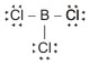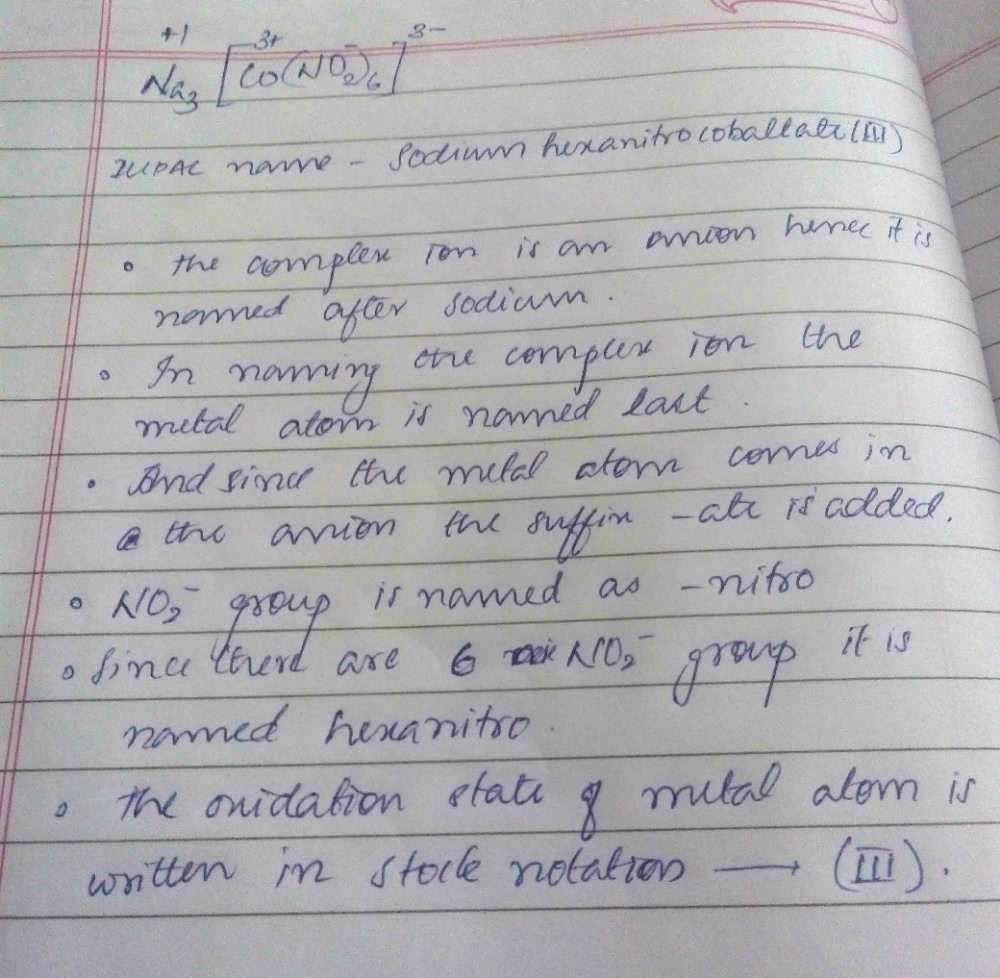Courses

# NEET UG Chemistry Mock Test 2

## 45 Questions MCQ Test NEET Mock Test Series & Past Year Papers | NEET UG Chemistry Mock Test 2

Description
This mock test of NEET UG Chemistry Mock Test 2 for NEET helps you for every NEET entrance exam. This contains 45 Multiple Choice Questions for NEET NEET UG Chemistry Mock Test 2 (mcq) to study with solutions a complete question bank. The solved questions answers in this NEET UG Chemistry Mock Test 2 quiz give you a good mix of easy questions and tough questions. NEET students definitely take this NEET UG Chemistry Mock Test 2 exercise for a better result in the exam. You can find other NEET UG Chemistry Mock Test 2 extra questions, long questions & short questions for NEET on EduRev as well by searching above.
QUESTION: 1

### Chloroform, on heating with zinc dust and water, forms

Solution: When chloroform is refluxed with zinc dust and water it gives methane. Zn+2 H2O ----- Zn(OH) 2+ 2(H) and CHCl3+ 6(H) ------- CH4+ 3HCl
QUESTION: 2

Solution:
QUESTION: 3

### The three fundamental quantities are

Solution:
QUESTION: 4
Which one of the following is used in the preparation of styrene?
Solution:
QUESTION: 5
For the energy levels in an atom, which one of the following statements is correct?
Solution:
QUESTION: 6
The enzyme pepsin hydrolyses
Solution:
QUESTION: 7

BCl3 is a planar molecule because in this molecule boron is

Solution:

BCl3 - Boron Trichloride:BCl3 is electron deficient molecules having six electrons in the valence shell of their respective central atoms
To complete their octets, the central atom in each case can accept a pair of electrons from the chlorine atom of another molecule froming dimeric structures.
However, because of small size of B, it cannot accommodate four big sized Cl atoms around it.
Therefore, BCl3 prefers to exist as monomeric planar molecule in which B atom is sp2 hybridized

QUESTION: 8

The enthalpy of combustion of C(graphite ) and C (diamond) are -393.5 and -395.4 KJ mol⁻1 respectively .The enthalpy of conversion of C(graphite) to C (diamond) in KJ mol⁻1 is

Solution:
QUESTION: 9
Which of the following factors will favour the reverse reaction in a chemical equilibrium?
Solution:
QUESTION: 10
The order of a reaction depends upon
Solution:
QUESTION: 11

If one mole of an ideal gas at 27oC temperature is compressed reversibly from 20 litres to 10 litres, then work done during the process is (R=8.314J-K⁻1-mol⁻1)

Solution:
QUESTION: 12
Which of the following ingredients is not used in sun burn creams?
Solution:
QUESTION: 13
Which of the following molecules contains asymmetric carbon atom ?
Solution:
QUESTION: 14
The name of the element with atomic number 100 was adopted in honour of
Solution: Fermium (symbol Fm and atomic number 100) was named for the American physicist Enrico Fermi. It is a synthetic, radioactive, metallic transuranic element of the actinide series.
QUESTION: 15

IUPAC name of Na₃[Co(NO₂)₆] is

Solution:Hence D is correct.

QUESTION: 16

The equilibrium in the reaction Cr₂O₇2⁻ → 2CrO₄2⁻ is shifted to right in

Solution:
QUESTION: 17
Reaction taking place at anode is
Solution:
QUESTION: 18
Which of the following is the best source of thallium?
Solution:
QUESTION: 19
The compressibility factor of an ideal gas is
Solution:
QUESTION: 20
Microcosmic salt is
Solution:
QUESTION: 21
Which one of the following is not a use of potash alum?
Solution:
QUESTION: 22
A pseudo solid is
Solution:
QUESTION: 23
The most stable hydride is
Solution:
QUESTION: 24

Which of the following does not show hydrogen bonding?

Solution:
QUESTION: 25
Which of the following is most volatile?
Solution: HF is a gas and due to presence of hydrogen bonding HCl is a liquid so,it is more volatile. Due to high electronegative nature HF is ionic in nature and HCl has covalent bonding this is also the reason why HF is least volatile than HCl.
QUESTION: 26
Which of the following alkaline earth metal is most abundant in the earth's crust?
Solution:
QUESTION: 27
The double bond in an alkene
Solution:
QUESTION: 28
Water softening by Clarke's process uses
Solution:
QUESTION: 29
Neutralization of an acid with a base, invariably results in the production of
Solution:
QUESTION: 30
Which is the chiral molecule?
Solution:
QUESTION: 31
Which of the following compound will make precipitate most readily with AgNO₃?
Solution:
QUESTION: 32
If N and S elements are present in organic compounds, then during Lassaigne's test both change into
Solution:
QUESTION: 33
In its reaction with sodium, 1 mol of a compound X gives 1 mol of H₂. Which one of the following compounds might be X?
Solution:
QUESTION: 34
Which gives more stable product ?
Solution: The 1,2-addition has a smaller activation energy than 1,4-addition - it occurs faster than 1,4 addition, because the bromide nucleophile is closer to carbon #2 then to carbon #4. However, the 1,4-product ismore stable than the 1,2-product.
QUESTION: 35

Which of the following complex will show geometrical as well as optical isomerism?

Solution:
QUESTION: 36
Which of the following pair of elements belongs to same period of the periodic table?
Solution:
QUESTION: 37
Which of the following is used for making seat covers and carpet fibres?
Solution:
QUESTION: 38
Aniline is usually purified by
Solution:
QUESTION: 39
In which of the following complexes, oxidation state of metal is zero ?
Solution:
QUESTION: 40
The limiting radius ratio for tetrahedral shape is
Solution:
QUESTION: 41
Which one of the following aqueous solution will have the lowest freezing point?
Solution:
QUESTION: 42
Which of the following has asymmetric carbon atoms?
Solution:
QUESTION: 43
4.4 g of an unknown gas occupies 2.24 litres of volume at standard temperature and pressure. The gas may be
Solution:
QUESTION: 44
Which of the following colloids are solvent-hating colloids?
Solution:
QUESTION: 45
AgCl is soluble in
Solution: